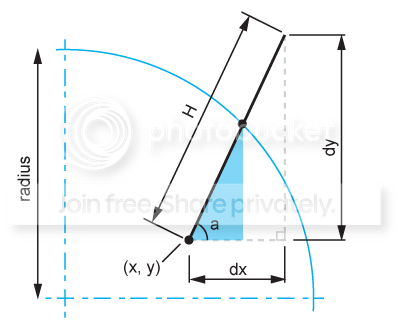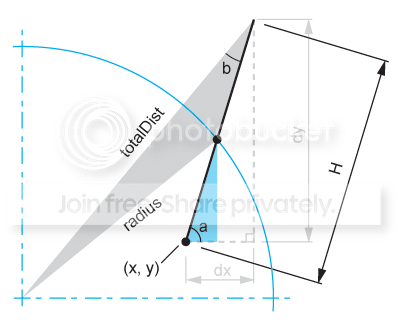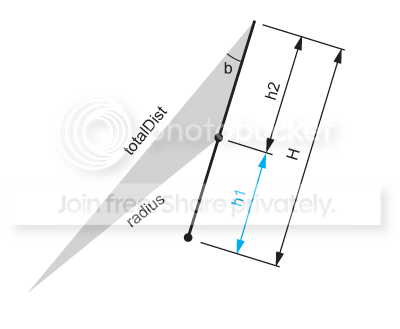A Flash Developer Resource Site

1. ## Tricky trigonometry question

OK, This one really needs a diagram to explain so check the attached image I created.
(I need to find the lengths of the blue triangle).A car is sitting at a known point within the perimeter of a circle of a known radius.
It travels in a straight line in a known direction for a known distance which happens to take it outside the circle.

How do I calculate the coordinate for the point at which the car intersects the circle?

Two more minor details...
1) The car does not necessarily begin at the center of the circle.
2) The direction the car takes does not necessarily radiate from the center either.Reply With Quote

2. It doesn't matter where the car starts from. Wherever the car intercepts the circle, the distance between the car and the centre of the circle is the radius. To get the point of intersection all you then need is the angle between the centre of the circle and the car's position.

So, function for getting the angle between 2 points (in radians):
Code:
```function getAngle(x:Number, x2:Number, y:Number, y2:Number):Number {
return Math.atan2(y-y2, x-x2);
}```
So to get the point of interception:
Code:
```var a:Number = getAngle(car._x, circleCentreX, car._y, circleCentreY);
var x:Number = Math.cos(a) * radius + circleCentreX;
var y:Number = Math.sin(a) * radius + circleCentreY;```
Obviously put your own variables in there.Reply With Quote

3. Doesn't seem like the last reply really addressed the issue - don't think the OP really knows the position where the car intersects the circle. (IE doesn't know the values of car._x, car._y).

Denote vector quantities with []. You can solve the problem by writing the position of the car in parametric form:

[C(t)] = [C0] + [Cdir]*t

[C(t)] is cars position at time t.
[C0] is cars position at t=0 (denoted x,y in your original figure)
[Cdir] is a unit vector that points in the direction of the cars motion (denoted dx,dy in your figure, but you must normalize it)

You are looking for the time at which the distance between the car and the center of the circle (denoted [B]) is R. Or equivalently, when the distance^2 is equal to R^2:

dot( [C0]-[B]+[Cdir]t, [C0]-[B]+[Cdir]t) = R*R

This is a quadratric equation in t. Solve for t using quadratic formula:

t = (-b +/- sqrt(bb-4ac) ) / 2a

where:
a = 1
b = 2 * dot( [C0]-[B], [Cdir] )
c = dot([C0]-[B],[C0]-[B]) - R*R
(ie the distance^2 between cars start and circles center, minus R*R)

There will be two roots for t - pick the one that's positive. (The negative root indicates the time the car intersected the circle as it entered it in the past). Once you know t, you know the position [C(t)] at the time of intersection. You can plug those numbers into dudeqwerty's solution.Reply With Quote

4. beat me! that was a fun one too, poopy.Reply With Quote

5. Heh, a pack of math nerds on the prowlTook 'er down like a limpy gazelle.Reply With Quote

6. Lmao, you guys are freaks!Thankyou!! I've been struggling with this for days.Reply With Quote

7. Okay fun's not over yet though. I was just considering if this can be done by using the rule of sines too? Here's what I mean...The dark gray triangle has two known sides and I think angle 'b' can be found too. So is that enough to find the third side of the triangle? If so then we can subtract that length from H and presto, blue triangle can be solved!

So Pythagora for totalDist:
PHP Code:
``` totalDist = Math.sqrt((x + dx) * (x + dx) + (y + dy) * (y + dy));  ```
and angle 'b' my trig is not so good but I'm pretty sure it could be done. Maybe find the top angle of the imaginary triangle just used in that last pythagora step above and subtract the top angle of the dotted line triangle (180 - (a + 90)).Reply With Quote

8. Would leave this to solve...Question 1) Is this way even possible or am I assuming too much?
Question 2) Even if it does work would it just take longer to do it this way anyway?Reply With Quote

9. That might work, although it will have the same branch cut issue as the parametric solution. Recalling vaguely from geometry, SAS (side-angle-side) SSS and ASA all uniquely specify a triangle, but SSA does not. The figure you drew has this SSA arrangement and your triangle is not unique. I can make a second triangle with the same SSA - by picking the same other point, behind the car.

Not to say you couldn't tackle the problem going that way - always more than one way to get the job done. Wikipedia's article on the law of sines appears to have a special section on this, entitled "the ambiguous case".Reply With Quote

10. Seems to me the question was:
How do I calculate the coordinate for the point at which the car intersects the circle?
And seeing as he can calculate totalDist, how does he not know what car_.x and car._y are?

Am I missing something?Reply With Quote

11. @dudeqwerty: one of the OP's original conditions is that the car's direction does not necessarily radiate from the circle's center.Reply With Quote

12. rachil0: Thanks for all your info. Did some research on "the ambiguous case" and you're certainly right.dudeqwerty: Not sure I follow you. totalDist just gives the distance to the point outside the circle, which isn't what I'm chasing.Reply With Quote

13.Reply With Quote

angle, distance, radius, trig, trigonometry####Posting Permissions

• You may not post new threads
• You may not post replies
• You may not post attachments
• You may not edit your posts
•

 » Home » Movies » Tutorials » Submissions » Board » Links » Reviews » Feedback » Gallery » Fonts » The Lounge » Sound Loops » Sound FX » About FK » Sitemap